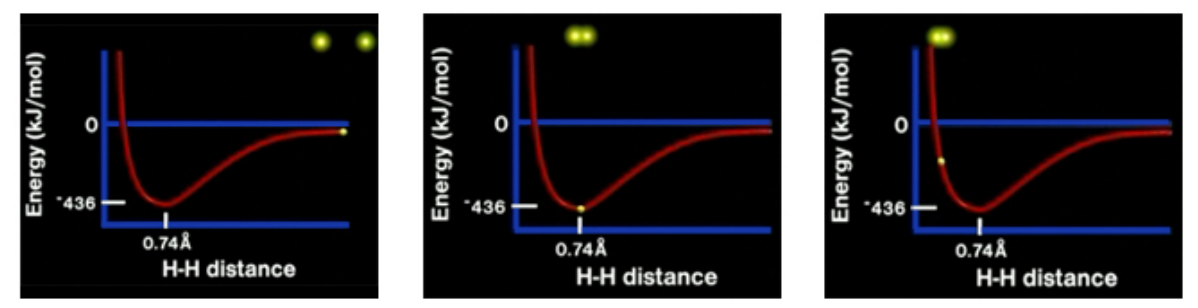# Problem: Part A. In the video, you can see that when two hydrogen atoms approach one another, a covalent bond is formed due to the overlap of the 1s orbital of each hydrogen atom. The process by which a covalent bond is formed is explained with the help of the graph of potential energy versus the distance between the two atoms. Identify which of the following statements are correct.When two hydrogen atoms that are far apart approach each other, the potential energy decreases. At a distance of 0.50 Å the potential energy is less than that at 0.74 Å. When the potential energy is zero, a covalent bond is formed. When the distance between two hydrogen atoms is 0.74 Å, a covalent bond is formed. When two hydrogen atoms are very far apart, the potential energy approaches zero.Check all that apply.

###### FREE Expert SolutionView Complete Written Solution
###### Problem Details

Part A. In the video, you can see that when two hydrogen atoms approach one another, a covalent bond is formed due to the overlap of the 1s orbital of each hydrogen atom. The process by which a covalent bond is formed is explained with the help of the graph of potential energy versus the distance between the two atoms. Identify which of the following statements are correct.

When two hydrogen atoms that are far apart approach each other, the potential energy decreases.

At a distance of 0.50 Å the potential energy is less than that at 0.74 Å.

When the potential energy is zero, a covalent bond is formed.

When the distance between two hydrogen atoms is 0.74 Å, a covalent bond is formed.

When two hydrogen atoms are very far apart, the potential energy approaches zero.Check all that apply.